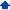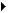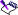### Working with VBA methods

You can use VBA to get system information, such as the system’s date and time values. Retrieving the system’s date and time values using VBA is very easy because all of the Windows functions are hidden, and all you have to do is call the appropriate VBA method.

#### To access the system’s date and time values1 Click ToolsVisual BasicVisual Basic Editor.
 2 Double-click Project(Document1) in the Project view.
 3 Double-click the WordPerfect objects folder in the Project view.
 4 Double-click ThisDocument, and type the following lines of code in the Editor box:
 `Public Sub ShowTime()`
 `End Sub`
 This code will add a new method to the ThisDocument class. Make sure that the ShowTime method is declared public so that you can access this macro from WordPerfect.
 5 Type the following new code in the ShowTime method to declare two new variables:
 `Public Sub ShowTime()`
 `Dim myTime`
 `Dim myDate As Date`
 `End Sub`
 6 Type the following new code in the ShowTime method to populate the myTime and the myDate variables:
 `Public Sub ShowTime()`
 `Dim myTime`
 `Dim myDate As Date`
 `myTime = Time`
 `myDate = Date`
 `End Sub`
 7 Type the following new code in the ShowTime method to convert the two variables to string data types:
 `Public Sub ShowTime()`
 `Dim myTime`
 `Dim myDate As Date`
 `myTime = Time`
 `myDate = Date`
 `Dim myStrTime, myStrDate, Msg As String`
 `myStrDate = Str(myDate)`
 `myStrTime = Str(myTime)`
 `End Sub`
 8 Type the following new code in the ShowTime method to populate and display the Msg variable:
 `Public Sub ShowTime()`
 `Dim myTime`
 `Dim myDate As Date`
 `myTime = Time`
 `myDate = Date`
 `Dim myStrTime, myStrDate, Msg As String`
 `myStrDate = Str(myDate)`
 `myStrTime = Str(myTime) `
 `Msg = “The date is ” & myStrDateI & “ and the time is ” & myStrTime`
 `MsgBox Msg`
 `End Sub`• For the purpose of this procedure, a message box was selected to display the date and time (myDate, myTime, myStrDate, and myStrTime); however, you can create a new form and display the time and date in this form. For more information about VBA, see Microsoft Visual Basic Help in the Visual Basic Editor.